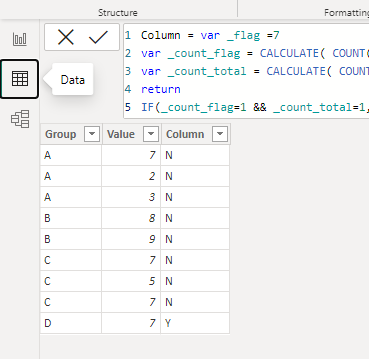cancel
Showing results for
Did you mean:Helper III

## Using DAX I want to create a flag off of a group if a specific value in another column appears once.

I would like to flag a group if only 7 in value appears once.

 Group Value A 7 A 2 A 3 B 8 B 9 C 7 C 5 C 7 D 7

I would like it to look like. Note that only D has 7 appear once. It is the only acceptable outcome.

 Group Value Result A 7 N A 2 N A 3 N B 8 N B 9 N C 7 N C 5 N C 7 N D 7 Y
1 ACCEPTED SOLUTIONCommunity Support

Hi , @user35131

Here are the steps you can refer to :
If you want to add a calculated column in table , you can click "New Column" and enter:

``````Column = var _flag =7
var _count_flag = CALCULATE( COUNT('Table'[Value]) ,'Table'[Value]=_flag,ALLEXCEPT('Table','Table'[Group]))
var _count_total = CALCULATE( COUNT('Table'[Value]) ,ALLEXCEPT('Table','Table'[Group]))
return
IF(_count_flag=1 && _count_total=1,"Y","N")``````If you want to add a measure on the visual , you can click "New Measure" and enter this:

``````Measure = var _flag =7
var _cur_group=MAX('Table'[Group])
var _count_flag =COUNTROWS( FILTER(ALLSELECTED('Table') , 'Table'[Group] = _cur_group && 'Table'[Value]=_flag))
var _count_total =COUNTROWS( FILTER( ALLSELECTED('Table')  , 'Table'[Group] =_cur_group))
return
IF(_count_flag=1 && _count_total=1 ,"Y","N")``````If this method does not meet your needs, you can provide us with your special sample data and the desired output sample data in the form of tables, so that we can better help you solve the problem.

Thank you for your time and sharing, and thank you for your support and understanding of PowerBI!

Best Regards,

Aniya Zhang

If this post helps, then please consider Accept it as the solution to help the other members find it more quicklyCommunity Support

Hi , @user35131

Here are the steps you can refer to :
If you want to add a calculated column in table , you can click "New Column" and enter:

``````Column = var _flag =7
var _count_flag = CALCULATE( COUNT('Table'[Value]) ,'Table'[Value]=_flag,ALLEXCEPT('Table','Table'[Group]))
var _count_total = CALCULATE( COUNT('Table'[Value]) ,ALLEXCEPT('Table','Table'[Group]))
return
IF(_count_flag=1 && _count_total=1,"Y","N")``````If you want to add a measure on the visual , you can click "New Measure" and enter this:

``````Measure = var _flag =7
var _cur_group=MAX('Table'[Group])
var _count_flag =COUNTROWS( FILTER(ALLSELECTED('Table') , 'Table'[Group] = _cur_group && 'Table'[Value]=_flag))
var _count_total =COUNTROWS( FILTER( ALLSELECTED('Table')  , 'Table'[Group] =_cur_group))
return
IF(_count_flag=1 && _count_total=1 ,"Y","N")``````If this method does not meet your needs, you can provide us with your special sample data and the desired output sample data in the form of tables, so that we can better help you solve the problem.

Thank you for your time and sharing, and thank you for your support and understanding of PowerBI!

Best Regards,

Aniya Zhang

If this post helps, then please consider Accept it as the solution to help the other members find it more quickly